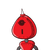# 6. Find the effective rate of interestif the nominal rate of interest is10% pa. and interest is calculatedhalf y

6. Find the effective rate of interest
if the nominal rate of interest is
10% pa. and interest is calculated
half yearly is​

### 1 thought on “6. Find the effective rate of interest<br />if the nominal rate of interest is<br />10% pa. and interest is calculated<br />half y”

1.Step-by-step explanation:

ҒϴᎡ ͲᎻᏆՏ ͲᎪᏦᎬ 100 ᎪՏ ᏢᎡᏆΝᏟᏆᏢᎪᏞ

Ꭱ = 10 % ᎪΝᎠ ᎻᎪᏞҒ ᎽᎬᎪᎡᏞᎽ ᎡᎪͲᎬ ᏔᏆᏞᏞ ᏴᎬ 5 %

5+5+0.25 = 10.25 %